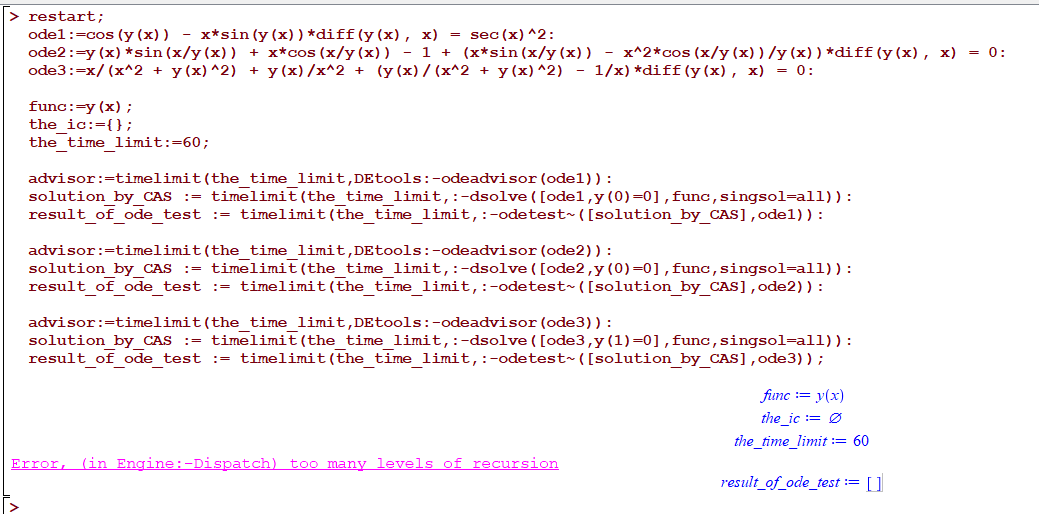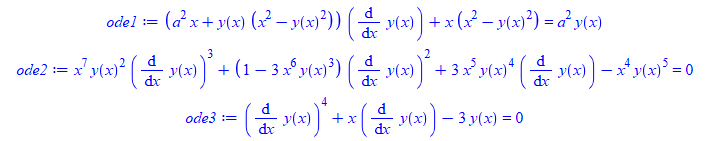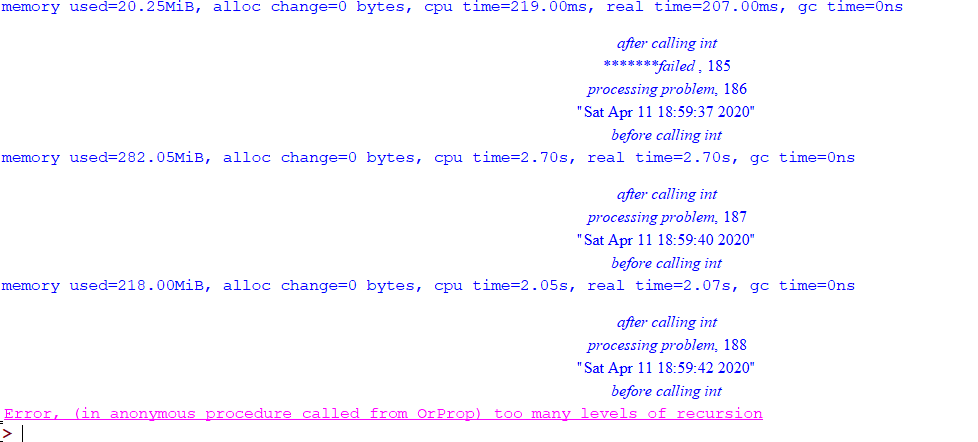## Maple 2020 doesn't start on Mac...

So, here again, I'm still having this problem with Maple 2020 on Machbook OS 10.13.6 (all updates have been performed): Maple 2020 does not start. I see an icon appearing in the dock but it then disappears soon without showing anything like splash something. I reinstalled and restarted the copmuter several times, reactivated the software several times (always successful), and still I'm having the problem. I installed java and then it worked for one time, but after that the same problem happens and I'm still having this issue.

I went through steps suggestged in the help and arrived at JAVA reisntallation. What else can I try?

Hiro

## Maple 2020 doesn't launch......

Suddently Maple 2020 (on Macbook) stops launching... I reinstalled it and restarted a couple of times.

Can someone give me some clues about what is causing this problem.

I really need it right now...

Thanks,

Hiro

## Maple freezes/can't open previously saved document...

Hello,

I have a particular problem that I haven't managed to find the solution to by googling.

9/10 times that I open Maple 2020 I can't seem to open any of my saved documents. it opens the start tab, and I'm still able to open a new document or worksheet, but then when I try to change to text instead of math, it won't let me. I can type, but I can't press backspace or enter.

I have tried restarting and shutting down my computer, but every time I have to just force quit Maple because it won't respond half of the time and it won't quit normally.

I'm running the latest version of Maple and using macOS Catalina version 10.15.5.

## how to enable autoscrolling in worksheet mode?...

https://www.mapleprimes.com/questions/203628-Maple-2015-No-Longer-Autoscroll-As

And according to the reply there at the time, this is supposed to have been fixed in Maple 2015.1:

It appears that the scrolling issue has been fixed in the next version of Maple

But ever since then, whenever I use Maple, the worksheet do not auto-scroll as the program runs and prints outout to the screen. I have to keep using the mouse to move the bar down to see the latest prints.

Not only that, the bar itself (on the right side) shrinks in size as more outpout is printed.

It becomes smaller and smaller with time, and becomes very hard to grab it with the mouse since it become so thin.  Once all the output is deleted, the bar become long again.

So I think there is an option to enable autoscrolling somewhere.

But I am not able to find it. I looked at options, display, general and interface and see nothing there to turn it on.

Sometimes I get the feeling that the folks at Maplesoft do not use Maple interface the way we users use it, becuase this is such a basic interface problem and any one who uses Maple interface for more than one day must see these problems all the time.

So how come these are still not fixed?  Here is a screen shot showing the bar on the right after long print out, and showing how it was before.I use only worksheet mode, not document mode. On windows 10.

## random error Error, (in Engine:-Dispatch) too many...

it took a while to track this. When I run these 3 ode's in this sequence, Maple gives

Error, (in Engine:-Dispatch) too many levels of recursion

and it has to be this sequence.  I also found I might have to run the code 2 or 3 times to get this error if it does not show first time.

Will show a movie below also.

Here is the code

```restart;
ode1:=cos(y(x)) - x*sin(y(x))*diff(y(x), x) = sec(x)^2:
ode2:=y(x)*sin(x/y(x)) + x*cos(x/y(x)) - 1 + (x*sin(x/y(x)) - x^2*cos(x/y(x))/y(x))*diff(y(x), x) = 0:
ode3:=x/(x^2 + y(x)^2) + y(x)/x^2 + (y(x)/(x^2 + y(x)^2) - 1/x)*diff(y(x), x) = 0:

func:=y(x);
the_ic:={};
the_time_limit:=60;

solution_by_CAS := timelimit(the_time_limit,:-dsolve([ode1,y(0)=0],func,singsol=all)):
result_of_ode_test := timelimit(the_time_limit,:-odetest~([solution_by_CAS],ode1)):

solution_by_CAS := timelimit(the_time_limit,:-dsolve([ode2,y(0)=0],func,singsol=all)):
result_of_ode_test := timelimit(the_time_limit,:-odetest~([solution_by_CAS],ode2)):

solution_by_CAS := timelimit(the_time_limit,:-dsolve([ode3,y(1)=0],func,singsol=all)):
result_of_ode_test := timelimit(the_time_limit,:-odetest~([solution_by_CAS],ode3));
```And here is a movie (this time it happend on second run) sometimes it happens on first run. so it is random.Maple 2020.1

Why does it happen and is there a work around?

## few errors using dsolve and and odetest...

Noticed few errors generated on some ode's. I do not know if these are expected or known, I thought may be someone might want to check them.

```restart;
ode1:=(a^2*x+y(x)*(x^2-y(x)^2))*diff(y(x),x)+x*(x^2-y(x)^2) = a^2*y(x);
ode2:=x^7*y(x)^2*diff(y(x),x)^3+(1-3*x^6*y(x)^3)*diff(y(x),x)^2+3*x^5*y(x)^4*diff(y(x),x)-x^4*y(x)^5 = 0;
ode3:=diff(y(x),x)^4+x*diff(y(x),x)-3*y(x) = 0;
````sol:=timelimit(60,:-dsolve(ode1,y(x))):`

Error, (in dsolve) numeric exception: division by zero

```sol:=timelimit(60,:-dsolve(ode2,y(x))):
timelimit(60,:-odetest([sol],ode2))
```

Error, (in IntegrationTools:-Indefinite:-AlgebraicFunction) argument is not an algebraic

```sol:=timelimit(60,:-dsolve(ode3,y(x))):
timelimit(60,:-odetest(sol,ode3));
timelimit(60,:-odetest(sol,ode3))
```

Error, (in odetest) received not an ODE w.r.t x(_T)
Error, (in odetest) received not an ODE w.r.t y(_T)

This last one is only due to how the solution is given. So I do not think it is a real problem.

Maple 2020.1, Physics 708

windows 10

Maple Worksheet - Error
Failed to load the worksheet /maplenet/convert/check.mw .

## Compiler package setup...

Error, (in Compiler:-Setup) unable to write to initialization file, C:\Program Files\Maple 2020\bin.X86_64_WINDOWS/launch.ini, possibly due to insufficient permissions
NULL;

when choose bat file

but i see sufficient permission

any w should i s

choose a particular compiler or even visual studio i have in my system

where can i find if anything else

## Error importing a big Excel data file xlsx...

Hello,

I try import a big Excel file with the first row as column labels for performing statistical etc.

I use this command :

data := Import(FileTools:-JoinPath(["d:", "directory", "subset2.xlsx"], platform = "windows"))

i need a dataframe for performing statistical with column label as first row, but i have this error :

Error, (in Import) invalid input: MatrixToDataFrame uses a 3rd argument, columns, which is missing

I can not understand what is this error...so, what i can do ?

Thanks, regards

## Can these vector space examples be explained?...

The uploaded worksheet contains two examples of the use of VectorCalculus[VectorSpace]. The first example seems explicable but the second does not.

I have tried and failed to find a full, clear explanation of how a vector describing a simple vector, spacecurve, or surface in the default vector space is transformed to appear in a user defined vector space.

Can anyone direct me to such an explanation, so that I can understand Maple's processing within the uploaded worksheet and enable me to use this Maple feature to future advantage?

VectorSpaceTest.mw

## Guidelines on how to use Grid:-Seq...

Hello

I wonder if someone on the list can give me some guidelines on how to use Grid:-Seq.

I have applied Grid:-Set and Grid:-Seq to one of my problems where parallelization is a possibility.   In this problem, I have a massive list of lists that needs to be processed in chunks and then a new list is returned.

What I've found so far:

1) Grid-Seq won't work if I use a procedure from a private module that calls local (or exported) functions of the same module.  Solution:  I need to use savelib (there is a bug in Grid:-Seq)

2) Grid-Seqs seems to be working fine up to a point where it simply stops.  For example:   Starting with 431895 sets, the procedure divides them into 864 sets of 500 elements (the last one does not usually contain 500 elements).  Then I use res:=Grid:-Seq['tasksize'=9](myFunction(newSets[i],...,....),i=1..864).   The messages sent out by Grid:-Seq are

Seq: using tasksize=9; number of elements= 864, number of partitions = 96

......

Seq: done sending all partitions

Seq: received results from all nodes; collating results

After two hours running and using 36 cores, the result is available in res.  The next step is to use the results in res for another cycle of calculations.  1503462 sets = 3007 sets of 500 elements.  The messages sent out by Grid:Seq are

Seq: using tasksize=9; number of elements= 3007, number of partitions = 335

......

Seq: done sending all partitions

The last message showed up on the screen after an hour. During the process, I saw the current tasks finishing and new ones starting.  However I could not see "Seq: received results from all nodes; collating result" after a day running.  I am using timelimit to be sure that all calculations will finish no matter what.

I have checked the system information and found that: 1) all 72 cores are running but they are jumping from 0% to 100% (in the example that works I could see all of them in 100% solid), 2) Memory is at 156 GB (200 Gb is the limit) and no swap to disk.

I have also noticed:

1. If I use Maple 2017, not even the first example works.
2. tasksize=9 somehow helps with similar problems.  If the size is left for Maple to decide, it seems that the problem happens for sets of smaller size.
3. On linux I had to use "kill -9" to remove maple from every single core. My impression is that Grid:-Seq does not clean up the processes after they finished.
4.  The problem seems to be on "results from all nodes; collating result".

I know that the explanation above in rather vague, but if someone has any clue or guidelines on how to solve this problem, please share it with me.

Many thanks

Ed

## partial differential equation ...

Hello,

is it possible to solve the attached partial differential equation with zero initial boundary condition?

If not by assuming diff(u(sigma, tau, phi, t), t, t)=0 is there an answer for the equation?

Thanks

lapla.mw

## Error, (in OrProp) too many levels of recursion. t...

This is very strange problem, and it seems to happen at random times.

From my worksheet, I call a function in a local fille maple_proc.mpl. This function ends up calling int() command with a timeout.

I Have the int() call wrapped by try...catch:.. end try in order to catch timeout and any other error.

Yet, when I run my worksheet, looping over hundreds of integrals, for each one, calling the function in maple_proc to integrate it, and after running for many many integrals, then sometimes, the worksheet terminates with error, at some iteration with error

Error, (in OrProp) too many levels of recursion |maple_proc.mpl:45|

So the try/catch I have in place are not catching the above error, wherever it is coming from.

line 44 in maple_proc.mpl, just does this (it is the line with cpu_time := .... below

```try
print(`before calling int`);
cpu_time := timelimit(180,CodeTools[Usage](assign('result_of_int',int(lst[i,1],lst[i,2])),output='realtime')):
print(`after calling int`);
catch "time expired":
#...
catch:
#...
end try;
```

Again, this works for sometimes for hundreds of iterations, and then on some entry, it gives this error.

I do not use recursion myself at all. i.e. I have no place where the function calls itself. Worksheet does a loop, and in each interation calls this function in maple_proc.mpl.

Sometimes, when I run the worksheet again, the error do not show up again. I have print statements in the catch above, and these do not show up at all, which means this error is not cought.

What should I do to find why this happens? This did not happen in Maple 2019.2. Is there any other way to trap this error so my loop does not terminate like this and I have to restart it again each time?

I am using Physics 642 and Maple 2020 on windows 10.

Update

I was able to strip all the code and make a MWE. There is a zip file attached.

one worksheet, which calls a maple_proc() function now in the same worksheet.

This function reads a plain text file in same folder, which contains list of integrals. The function maple_proc() does a "read" which reads all problems into a variable called lst

Now it simply does a LOOP over all entries in lst calling int() on each integral (one integral per line) with a timeout. You see there is catch() there.

I get the erorrAt iteration number 188 each time.

I will attach a ZIP file now, which contains one folder which contains the worksheet and the input plain textfile. All what you need to do to reproduce this is open the worksheet and evaluate the call there. You might want to edit the hardcoded folder in the worksheet to your own folder where you unzipped the zip file.

```currentdirName :="C:/MAPLE_BUG";
currentdir(currentdirName);
```

I hope someone will be able to repoduce this. It looks like a real bug in Maple 2020.

The problem is not in the integral 188 itself. Because if the loop starts at say 100 or 120, instead from 1, the integral now is processed OK and no error is generated.

MAPLE_BUG.zip

﻿